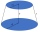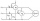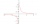# Cube in a sphere

The cube is inscribed in a sphere with volume 9067 cm3. Determine the length of the edges of a cube.

Result

a =  14.9 cm

#### Solution:

$V = 9067 \ cm^3 \ \\ V = \dfrac{ 4 }{ 3 } \pi r^3 \ \\ \ \\ r = \sqrt{ \dfrac{ 3 \cdot \ V }{ 4 \pi } } = \sqrt{ \dfrac{ 3 \cdot \ 9067 }{ 4 \cdot \ 3.1416 } } \doteq 12.9358 \ cm \ \\ \ \\ D = 2 \cdot \ r = 2 \cdot \ 12.9358 \doteq 25.8715 \ cm \ \\ \ \\ u = D = 25.8715 \doteq 25.8715 \ cm \ \\ \ \\ u = \sqrt{ 3 } a \ \\ \ \\ a = u/\sqrt{ 3 } = 25.8715/\sqrt{ 3 } \doteq 14.9369 = 14.9 \ \text { cm }$

Leave us a comment of this math problem and its solution (i.e. if it is still somewhat unclear...):

Showing 1 comment:Math student
i am good at this

1 year ago  1 Like#### Following knowledge from mathematics are needed to solve this word math problem:

Need help calculate sum, simplify or multiply fractions? Try our fraction calculator. Do you want to convert length units? Tip: Our volume units converter will help you with the conversion of volume units. Pythagorean theorem is the base for the right triangle calculator.

## Next similar math problems:

1. The hemisphereThe hemisphere container is filled with water. What is the radius of the container when 10 liters of water pour from it when tilted 30 degrees?
2. Secret treasureScouts have a tent in the shape of a regular quadrilateral pyramid with a side of the base 4 m and a height of 3 m. Determine the radius r (and height h) of the container so that they can hide the largest possible treasure.
3. Heptagonal pyramidA hardwood for a column is in the form of a frustum of a regular heptagonal pyramid. The lower base edge is 18 cm and the upper base of 14 cm. The altitude is 30 cm. Determine the weight in kg if the density of the wood is 0.10 grams/cm3.
4. Frustum of a coneA reservoir contains 28.54 m3 of water when completely full. The diameter of the upper base is 3.5 m while at the lower base is 2.5 m. Determine the height if the reservoir is in the form of a frustum of a right circular cone.
5. Cylinder and its circumferenceIf the height of a cylinder is 4 times its circumference. What is the volume of the cylinder in terms of its circumference, c?
6. Faces diagonalsIf the diagonals of a cuboid are x, y, and z (wall diagonals or three faces) respectively than find the volume of a cuboid. Solve for x=1.2, y=1.7, z=1.45
7. Isosceles triangle 10In an isosceles triangle, the equal sides are 2/3 of the length of the base. Determine the measure of the base angles.
8. Before yesterdayHe merchant adds a sale sign in his shop window to the showed pair of shoes in the morning: "Today by p% cheaper than yesterday. " After a while, however, he decided that the sign saying: "Today 62.5% cheaper than the day before yesterday". Determine the nTwo wattmeters are connected to measuring power in a 3 phase balanced load. Determine the total power and power factor if the two wattmeters read 1000 watts each (1) both positive and (2) second reading is negative
10. A rhombusA rhombus has sides of length 10 cm, and the angle between two adjacent sides is 76 degrees. Find the length of the longer diagonal of the rhombus.
11. Medians in right triangleIt is given a right triangle, angle C is 90 degrees. I know it medians t1 = 8 cm and median t2 = 12 cm. .. How to calculate the length of the sides?
12. Sides of right angled triangleOne leg is 1 m shorter than the hypotenuse, and the second leg is 2 m shorter than the hypotenuse. Find the lengths of all sides of the right-angled triangle.
13. Coordinates of square verticesI have coordinates of square vertices A / -3; 1/and B/1; 4 /. Find coordinates of vertices C and D, C 'and D'. Thanks Peter.
14. Surface of the cylinderCalculate the surface of the cylinder for which the shell area is Spl = 20 cm2 and the height v = 3.5 cm
15. Compound interest 3After 8 years, what is the total amount of a compound interest investment of \$25,000 at 3% interest, compounded quarterly? (interest is now dream - in the year 2019)
16. Equation 23Find value of unknown x in equation: x+3/x+1=5 (problem finding x)
17. Geography testsOn three 150-point geography tests, you earned grades of 88%, 94%, and 90%. The final test is worth 250 points. What percent do you need on the final to earn 93% of the total points on all tests?